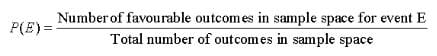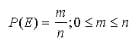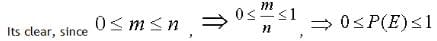# Statistics and Probability (Part - 3) Notes - GMAT

## GMAT: Statistics and Probability (Part - 3) Notes - GMAT

The document Statistics and Probability (Part - 3) Notes - GMAT is a part of GMAT category.
All you need of GMAT at this link: GMAT

Merits of Median
(i) Median is rigidly defined.
(ii) It can be easily understood and calculate.
(iii) The median is not much affected by extreme values and therefore it is a better representative as an average of given data.
(iv) The median can be calculated graphically, while mean can not be.
(v)  In some cases, median can be determined even by inspection.
(vi) If the class intervals are unequal then also median can be calculated.

Demerits of Median
(i) Median is not based on all the observations.
(ii) If the number of observations is even, median cannot be determined exactly.
(iii) If there is fluctuation of sampling then the median would be much affected by it.
(iv) It is not subject to algebraic treatment.

Uses of Median
(1) Since the median is middle term of an arrayed data, therefore it is the only average which is used while dealing with qualitative data which can be arrayed but cannot be measured quantitatively.
(2) Median is used for determining the typical value in problems concerning wages, distribution of wealth etc.

Mode
Mode of a given data is the value of that observation which occurs maximum number of times i.e. the observation which occurs with the highest frequency.

According to Croxton and Cowden, “The mode of a distribution is the value at the point around which, the items tend to be most heavily concentrated.”
Mode of ungrouped data
For a given ungrouped data, the mode can be located simply by inspection. It is variate which is having maximum frequency.
Empirical Formula: It two or more observations occurs the same number of time with highest frequency, then mode can be determined by following formula
Mode = 3 median – 2 mean

Merits of Mode:
(i) Mode can be easily understood and calculate.
(ii) It can be calculated graphically.
(iii) It is not affected by extreme values.
(iv) In some cases, it can be found by inspection also.
(v) It can be used for open ended distribution and qualitative data.

Demerits of Mode:
(i) Mode is not based upon all the observations.
(ii) Mode is ill-defined. It is not always possible to find a clearly defined mode.
(iii) Mode is affected to a greater extent by fluctuations of sampling.
(iv) Mode is not capable of further mathematical treatment. It is often indeterminate.

Uses of Mode
Mode is the average which is used to find the ideal size, e.g. in business forecasting, in manufacturing of ready-made garments, shoes etc.

PROBABILITY

HISTORY OF PROBABILITY
The theory of probability was originated from the games of chance related to gambling. An Italian Mathematician, Jerome Cardan (1501–1576) was the first to write a book named “Book on Games of Chance” published in 1663. Notable contributions were also made by mathematicians J. Bernoulli, P. Laplace and A. A. Markov. In the twentieth century, a book “Foundation of Probability” was published by Russian Mathematician Kolomogorov in 1933 and this was the first book to introduce probability as a set function.
SOME IMPORTANT OBJECTS

 (i) Coin : Coin is a well known object. It has two faces, one is Head and other is Tail. (ii) Die : A die is a well balanced solid cube having six faces marked with numbers (dots) from 1 to 6, one number of one face. The plural of die is dice. (iii) Playing Cards : A pack of playing cards contains 52 cards out of which 26 are red cards and 26 are black cards. These 52 cards are divided in four groups, each group is called a suit and has 13 cards. Name of these suits are: (i) Diamond (¨) (ii) Heart (©) (ii) Spades (ª) (iv) Club (§)

Out of these four suits Diamond and Heart are read cards and Spade and Club are black cards. Each suit having 13 cards which are 1, 2, 3,

...., 10, Jack, Queen and King. Card having 1 is also called an ace. Jack, Queen and King are known as face cards. Therefore total 12 face cards are in a pack of 52 playing cards.

SOME DEFINITIONS:
Experiment: An activity which ends in some well defined results is called an experiment. These results are called outcomes. There are two types of experiments:

(i) Deterministic experiment
Those experiments which when repeated under identical conditions produce the same results or outcomes are known as deterministic experiments.
Example: Formation of Methane in laboratory.

(ii) Random Experiment:
An experiment, when repeated under identical conditions do not produce the same outcome every time but the outcome is one of the several outcomes, it is known as Random Experiment:

Trial:
Performing an experiment once is called a trial.

Sample Space:
The collection of all the possible outcomes of a random experiment is called a sample space. It is usually denoted by S.
Example: After tossing a coin, possible outcomes are head and tail so sample space for tossing a coin consists of head and tail.
Event:
Each possible outcome of a trial is known as an event. It is generally denoted by E. It is of two types:
(i) Simple Event: If any event E contains only one outcome of sample space then it is known as simple event. In this way each outcome of sample space related to any experiment is a simple event.
Example: The experiment of throwing a die once consists of 6 simple events viz. coming the face showing up 1 or 2 or 3 or 4 or 5 or 6.
(ii) Compound Event: If any event contains more than one outcomes of sample space, then it is known as compound event.
Example: After throwing a die the outcome is an even number i.e. 2 or 4 or 6.

DIFFERENT APPROACHES TO PROBABILITY
There are following approaches to theory of probability:
(1) Empirical Approach
(2) Classical Approach
(3) Axiomatic Approach
Here we study only Empirical Approach to Probability

DEFINITION OF PROBABILITY
Let E be any event related to a Random experiment whose sample space has n outcomes and out of these n outcomes, the event can be performed by m outcomes, then probability of occurrence of event E will bei.e. probability of any event lies between 0 and 1.

Note:
(i) Probability of any event cannot be less than 0 and cannot be more than 1. So it can be any fraction from 0 to 1.
(ii) It p is the probability of occurrence of an event E and q is the probability of non occurrence of that event then
p + q = 1
q = 1 – p
(iii) The sum of the probabilities of all the possible outcomes of a trial is 1.

IMPOSSIBLE EVENT
If the number of favorable outcomes for an event is zero then the probability of occurrence of that event will be zero and such type of event is known as Impossible Event.

Sure Event or Certain Event
It the number of favorable outcomes for an event is equal to the total number of possible outcomes then the probability of occurrence of that event will be one and such type of event is known as sure or certain event.

The document Statistics and Probability (Part - 3) Notes - GMAT is a part of GMAT category.
All you need of GMAT at this link: GMATUse Code STAYHOME200 and get INR 200 additional OFF

Track your progress, build streaks, highlight & save important lessons and more!

,

,

,

,

,

,

,

,

,

,

,

,

,

,

,

,

,

,

,

,

,

;